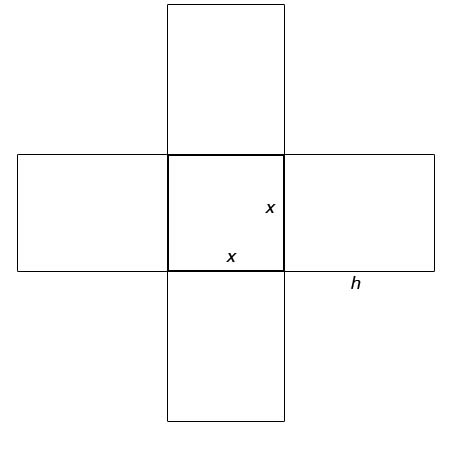SEARCH HOMEMath Central Quandaries & QueriesRita, a student: An open box with a square base is needed to have a volume of 10 cubic feet. (a) Express the amount A of material used to make such a box as a function of the length x of a side of the square base. (b) How much material is needed for a base 1 foot by 1 foot?Hi Rita,

Here is an approximate diagram for your problem:Let's let x be the sides of the square base and h be the height of the box. So for our formulae for our open box will be

 V=lwh 10 ft=(x)(x)(h) 10 ft=x2h The area of the base is (x)(x) and each of the four sides is (x)(h) A = 4xh + x2

Since the questions asks us to write the area in terms of one variable, x, you can manipulate the volume formula solving for h and substitute it into the area formula.

Hope this helps,

JaniceMath Central is supported by the University of Regina and The Pacific Institute for the Mathematical Sciences.